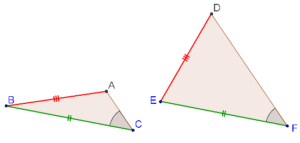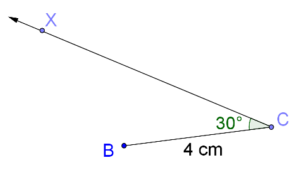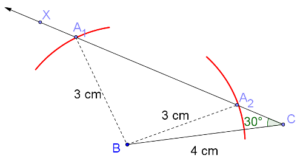# Is there an SSA Criterion?

Go back to  'Triangles-Quadrilaterals'

We have seen that the SAS criterion applies when two sides and the included angle of one triangle (respectively) equal the two sides and the included angle of another triangle. But what if the equality is between pairs of two sides and a non-included angle? Do we then have something like an SSA criterion?

Consider the following figure:In the two triangles ABC and DEF, we have:

1. AB = DE

2. BC = EF

3. ∠C = ∠F (non-included angles)

We see that even though two pairs of sides and a pair of angles are (correspondingly) equal, the two triangles are not congruent. Clearly, congruence does not hold through SSA, even though it does hold through SAS.

If you are still not convinced, let’s do an exercise. Suppose that there is a triangle two of whose sides have lengths 4 cm and 3 cm, and a non-included angle is 30°. Let’s try to geometrically construct such a triangle. If, during our construction process, we find that we can construct only one (unique) such triangle, then SSA congruence would be valid, but on the other hand, if we find that we can construct more than one such triangles, then SSA congruence would be invalid – because  then two different triangles can have the same two lengths and a non-included angle.

Here’s a step-by-step construction:

1. Construct BC = 4 cm.

2. Through C, draw a ray CX such that ∠BCX = 30°.3. Now, you have to take a point A on the ray CX such that AB = 3cm. How many locations of A are possible? Configure your compass such that the distance between its tip and the pencil’s tip is 3 cm. Now, place the tip of the compass on B, and you will find that you can mark off two points on CX:Thus, you have successfully constructed two different triangles (∆A1BC and ∆A2BC) given a pair of sides and a non-included angle. What does this mean?

1. A pair of sides and a non-included angle will not uniquely determine a triangle. In other words, congruence through SSA is invalid.
2. A pair of sides and the included angle will uniquely determine a triangle. In other words, congruence through SAS is valid.